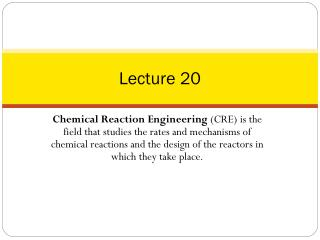# Lecture 20 - PowerPoint PPT PresentationDownload PresentationLecture 20

Lecture 20Download Presentation## Lecture 20

- - - - - - - - - - - - - - - - - - - - - - - - - - - E N D - - - - - - - - - - - - - - - - - - - - - - - - - - -
##### Presentation Transcript

1. Lecture 20 Chemical Reaction Engineering (CRE) is the field that studies the rates and mechanisms of chemical reactions and the design of the reactors in which they take place.

2. Last Lecture Energy Balance Fundamentals Substituting for

3. Web Lecture 20Class Lecture 16-Thursday 3/14/2013 • Reactors with Heat Exchange • User friendly Energy Balance Derivations • Adiabatic • Heat Exchange Constant Ta • Heat Exchange Variable Ta Co-current • Heat Exchange Variable Ta Counter Current

4. Adiabatic Operation CSTR Elementary liquid phase reaction carried out in a CSTR The feed consists of both - Inerts I and Species A with the ratio of inerts I to the species A being 2 to 1.

5. Adiabatic Operation CSTR • Assuming the reaction is irreversible for CSTR, A  B, (KC = 0) what reactor volume is necessary to achieve 80% conversion? • If the exiting temperature to the reactor is 360K, what is the corresponding reactor volume? • Make a Levenspiel Plot and then determine the PFR reactor volume for 60% conversion and 95% conversion. Compare with the CSTR volumes at these conversions. • Now assume the reaction is reversible, make a plot of the equilibrium conversion as a function of temperature between 290K and 400K.

6. CSTR: Adiabatic Example (exothermic) 1) Mole Balances:

7. CSTR: Adiabatic Example 2) Rate Laws: 3) Stoichiometry:

8. CSTR: Adiabatic Example 4) Energy Balance Adiabatic, ∆Cp=0

9. CSTR: Adiabatic Example Irreversible for Parts (a) through (c) (a) Given X = 0.8, find T and V (if reversible)

10. CSTR: Adiabatic Example Given X, Calculate T and V

11. CSTR: Adiabatic Example Given T, Calculate X and V (b) (if reversible)

12. CSTR: Adiabatic Example (c) LevenspielPlot

13. CSTR: Adiabatic Example (c) LevenspielPlot

14. CSTR: Adiabatic Example CSTR     X = 0.6     T = 360 K CSTR     X = 0.95     T = 395 K

15. CSTR: Adiabatic Example • PFR     X = 0.6 • PFR     X = 0.95

16. CSTR: Adiabatic Example - Summary

17. Energy Balance in terms of Enthalpy

18. PFR Heat Effects

19. PFR Heat Effects Need to determine Ta

20. Heat Exchange: Need to determine Ta

21. Heat Exchange Example: Case 1 - Adiabatic Adiabatic (Ua=0) and ΔCP=0 Energy Balance:

22. User Friendly Equations Constant Ta e.g., Ta = 300K B. Variable TaCo-Current C. Variable Ta Counter Current Guess Ta at V = 0 to match Ta0 = Ta0 at exit, i.e., V = Vf

23. Heat Exchanger Energy Balance Variable Ta Co-current Coolant Balance: In - Out + Heat Added = 0

24. Heat Exchanger Energy Balance Variable Ta Co-current In - Out + Heat Added = 0

25. Heat Exchanger – ExampleCase 1 – Constant Ta Elementary liquid phase reaction carried out in a PFR Heat Exchange Fluid Ta FA0 FI T The feed consists of both inerts I and species A with the ratio of inerts to the species A being 2 to 1.

26. Heat Exchanger – ExampleCase 1 – Constant Ta 1) Mole Balance: 2) Rate Laws:

27. Heat Exchanger – ExampleCase 1 – Constant Ta 3) Stoichiometry: • 4) Heat Effects:

28. Heat Exchanger – ExampleCase 1 – Constant Ta Parameters:

29. PFR Heat Effects Heat generated Heat removed

30. Heat Exchanger – ExampleCase 2 – Adiabatic Mole Balance: Adiabatic and ΔCP=0 Ua=0 Energy Balance: Additional Parameters (17A) & (17B)

31. Adiabatic PFR

32. Example: Adiabatic Find conversion, Xeq and T as a function of reactor volume Xeq X T rate X V V V

33. Heat Exchange Need to determine Ta

34. User Friendly Equations A. Constant Ta (17B) Ta = 300K Additional Parameters (18B – (20B): B. Variable TaCo-Current C. Variable Ta Countercurrent Guess Ta at V = 0 to match Ta0 = Ta0 at exit, i.e., V = Vf

35. Heat Exchange Energy Balance Variable Ta Co-current Coolant balance: In - Out + Heat Added = 0 All equations can be used from before except Taparameter, use differential Ta instead, adding mC and CPC

36. Heat Exchange Energy Balance Variable Ta Co-current In - Out + Heat Added = 0 All equations can be used from before except dTa/dV which must be changed to a negative. To arrive at the correct integration we must guess the Ta value at V=0, integrate and see if Ta0 matches; if not, re-guess the value for Ta at V=0

37. Derive the user-friendly Energy Balance for a PBR Differentiating with respect to W:

38. Derive the user-friendly Energy Balance for a PBR Mole Balance on species i: Enthalpy for species i:

39. Derive the user-friendly Energy Balance for a PBR Differentiating with respect to W:

40. Derive the user-friendly Energy Balance for a PBR Final Form of the Differential Equations in Terms of Conversion: A:

41. Derive the user-friendly Energy Balance for a PBR Final form of terms of Molar Flow Rate: B:

42. Reversible Reactions The rate law for this reaction will follow an elementary rate law. WhereKe is the concentrationequilibriumconstant. Weknow from Le Chaltlier’slaw that if the reaction is exothermic, Kewilldecrease as the temperature is increased and the reactionwill be shifted back to the left. If the reaction is endothermic and the temperature is increased, Kewillincrease and the reactionwillshift to the right.

43. Reversible Reactions Van’t Hoff Equation:

44. Reversible Reactions For the special case of ΔCP=0 Integrating the Van’t Hoff Equation gives:

45. Reversible Reactions Xe KP endothermicreaction endothermicreaction exothermicreaction exothermicreaction T T

46. End of Lecture 20